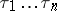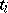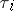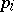# Arithmetization

(diff) ← Older revision | Latest revision (diff) | Newer revision → (diff)
The first arithmetization was constructed by K. Gödel  for the proof of the incompleteness of formal arithmetic (cf. Gödel incompleteness theorem). More precisely, Gödel put the letters of the alphabet in correspondence with some, pairwise different, natural numbers and attached to the wordthe number, whereis the number corresponding to the letter, andis the-th prime number in the natural number sequence. Such an enumeration is called a Gödel enumeration. In a broad sense every enumeration of words arising from arithmetization is called a Gödel enumeration and the number corresponding to a word is called its Gödel number.